You are using an outdated browser. Please upgrade your browser to improve your experience.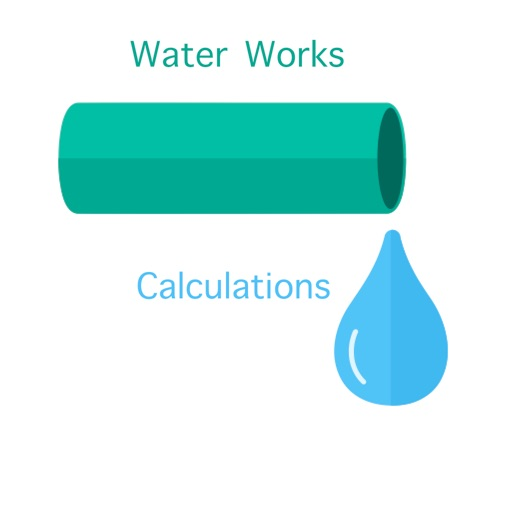## Fluid Flow Calculator : contains 94 Calculators for calculating flow in Weirs,Channels,Pipes,Water works and Civil Engineering parameters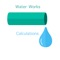# Waterworks Calculations : weirs,channels,pipes

by V PUGAZHENTHI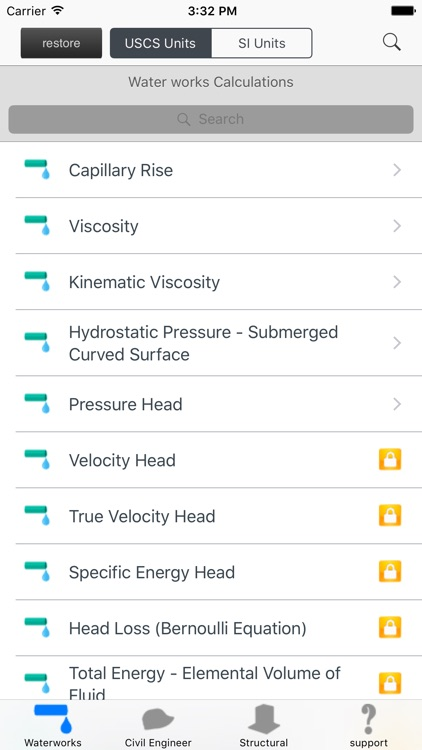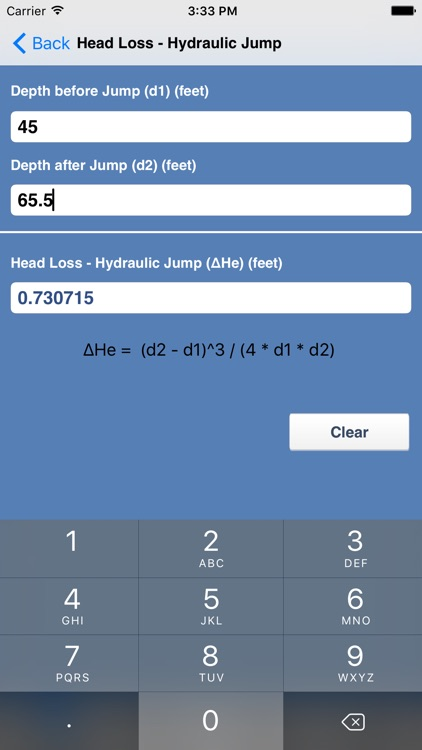### What is it about?

Fluid Flow Calculator : contains 94 Calculators for calculating flow in Weirs,Channels,Pipes,Water works and Civil Engineering parameters.### App Details

Version
1.0
Rating
NA
Size
20Mb
Genre
Education Reference
Last updated
July 18, 2016
Release date
July 18, 2016
More info

### Waterworks Calculations is FREE but there are more add-ons

• \$3.99

Unlock All the 96 Calculators

### App Screenshots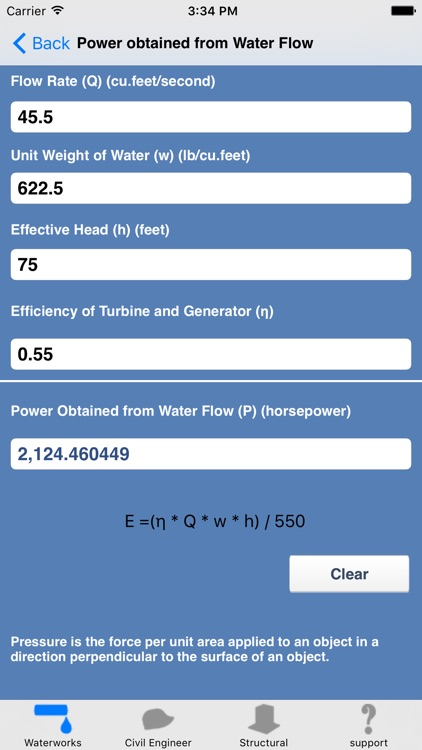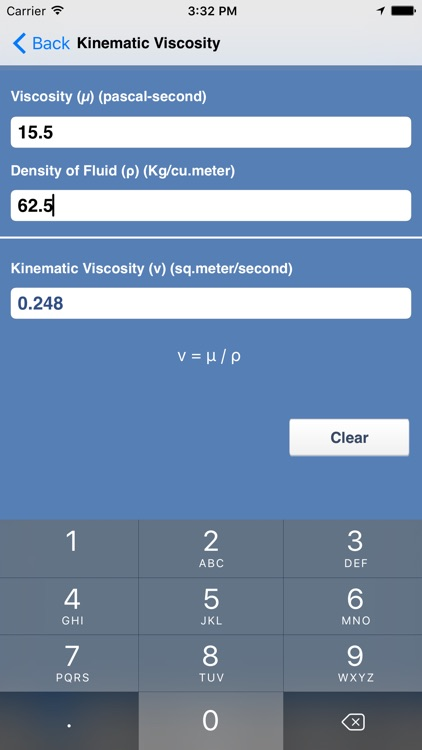### App Store Description

Fluid Flow Calculator : contains 94 Calculators for calculating flow in Weirs,Channels,Pipes,Water works and Civil Engineering parameters.
- Available in Metric ( SI ) and Imperial Units ( USCS )
• Capillary Rise
• Viscosity
• Kinematic Viscosity
• Hydrostatic Pressure
• Pressure Head
Offered as in-App purchase :
• Velocity Head
• True Velocity Head
• Specific Energy Head
• Head Loss (Bernoulli Equation)
• Total Energy - Elemental Volume of Fluid
• Total Head of Flowing Fluid
• Average Velocity of Flow
• Summation of all Forces (X direction)

• Froude Number
• Reynolds Number
• Weber Number
• Head Loss due to Friction
• Head Loss due to Friction (Darcy-Weisbach Formula)
• Chezy Velocity
• Chezy Coefficient

• Manning Flow Velocity
• Manning Flow Velocity using Pipe Diameter
• Flow Rate (Manning Formula)
• Head Loss due to Friction (Manning Formula)
• Pipe Diameter (Manning Formula)

• Mean Flow Velocity (Hazen-Williams)
• Mean Flow Velocity - Pipes Flowing Full (Hazen-Williams)
• Fluid Flow Rate (Hazen-Williams)
• Head Loss due to Friction (Hazen-Williams)
• Pipe Diameter (Hazen-Williams)

• Head Loss across a sudden enlargement of Pipe Diameter (Bernoulli Equation & Archer Equation)
• Head Loss due to Gradual Conical Enlargement
• Head Loss across a sudden contraction of Pipe
• Head Loss across a sudden contraction of Pipe (Brightmore Equation)
• Head Loss - Pipe Fittings

• Loss Coefficient
• Orifice Flow Rate
• Coefficient of Contraction
• Initial Velocity of Fluid Jets
• Gage Pressure at Tube Entrance (Conical Tubes)
• Velocity of Wave in a Pipe (Water Hammer)
• Internal Pressure in Pipe
• Stress on Pipe Material
• Section Factor
• Critical Depth of Flow - Open Channel Flow

• Force - Hydraulic Jump
• Rate of Change of Momentum - Hydraulic Jump
• Head Loss - Hydraulic Jump
• Stress on Pipe due to Temperature Change
• Movement in Length of Pipe due to Temperature Change
• Resultant Force on Bend in Pipe

• Elevation Difference between Headwater and Tailwater (Entrance and Exit Submerged)
• Velocity of Flow in Culvert (Entrance and Exit Submerged)
• Elevation Difference between Headwater and Tailwater (Entrance Submerged/Unsubmerged & Free Exit)
• Velocity of Flow in Culvert (Entrance Submerged/Unsubmerged & Free Exit)

• Flow Rate - Open Channel Flow
• Average Velocity - Rectangular Channels
• Critical Depth - Rectangular Channels
• Flow Rate - Rectangular Channels
• Discharge of Fluid per Unit Length - Rectangular Channels
• Minimum Specific Energy - Rectangular Channels

• Average Velocity - Triangular Channels
• Critical Depth - Triangular Channels
• Flow Rate - Triangular Channels

• Average Velocity - Parabolic Channels
• Critical Depth - Parabolic Channels
• Flow Rate - Parabolic Channels

• Average Velocity - Trapezoidal Channels
• Critical Depth - Trapezoidal Channels
• Flow Rate - Trapezoidal Channels
• Minimum Specific Energy - Trapezoidal Channels

• Area of Cross Section - Circular Channels
• Critical Depth - Circular Channels
• Top Width - Circular Channels
• Flow Rate - Circular Channels

• Flow Rate - Rectangular Weir
• Flow Rate - Triangular Weir (Notch Angle of 90 degrees)
• Flow Rate - Triangular Weir (Notch Angle of 60 degrees)
• Flow Rate - Triangular Weir (Notch Angle of 30 degrees)
• Flow Rate - Trapezoidal Weir
• Flow Rate - Broad Crested Weir

• Quantity of Sediment transported as Bed Load (Schoklitsch Formula)
• Evaporation Rate (Meyer Equation)
• Wind Factor
• Peak Discharge (Rational Formula)
• Rainfall Intensity (Steel Formula)

• Flow Rate - Groundwater (Darcy's Law)
• Fire Demand Rate
• Flow Rate - Gravity Well (Dupuit Formula)
• Flow Rate - Artesian Well
• Economic Pipe Diameter
• Flow Rate - Venturi Flow Meter
• Power obtained from Water Flow

Disclaimer:
AppAdvice does not own this application and only provides images and links contained in the iTunes Search API, to help our users find the best apps to download. If you are the developer of this app and would like your information removed, please send a request to [email protected] and your information will be removed.